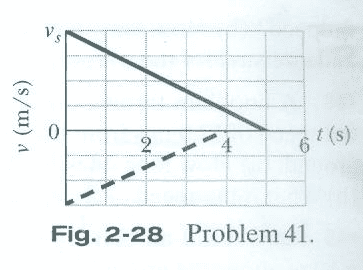# How to find the distance between two decelerating trains?

## Homework Statement

"As two trains move along a track, their conductors suddenly notice that the are headed toward each other. Figure 2-28 gives their velocities as functions of time t as the conductors slow th trains. The figure's vertical scaling is set by vs = 40.0 m/s. The slowing processes begin when the trains are 200 m apart. What is their separation when both trains have stopped?"## Homework Equations

x - x0 = v0t + ½at2
v0A = 40 m/s
aA = -0.8 m/s2
v0B = -30 m/s
aA = 0.75 m/s2

## The Attempt at a Solution

First, I listed all the equations for the movement of the trains.
##v_A(t) = -\frac{4}{5}t+40##
##s_A(t) = -\frac{2}{5}t^2+40t##
##v_B(t) = \frac{3}{4}t-30##
##s_B(t) = \frac{3}{8}t^2-30t##

Plugging in the appropriate times...
##s_A(5) = -10+200 = 190##
##s_B(4) = 6-120 = -114##

Adding them together, I do not get the answer listed in the book.
##s_A(5) + s_B(4) = 76 m ≠ 40m##

Though there are two very peculiar things I noticed with the velocity equations. When I set them to zero:
##0 = -\frac{4}{5}t+40##
##-40 = -\frac{4}{5}t##
##-40 = -\frac{4}{5}t##
##t = 50 s##

##0 = \frac{3}{4}t-30##
##30 = \frac{3}{4}t##
##t = 40s##

Those are clearly not the values depicted in Figure 2-28. I may be wrong, but these velocity equations may have two different constants. Anyway, the second thing; when I set both displacements to (4), I somehow end up with the correct answer, despite the fact that train A doesn't stop at 4 seconds.

##s_A(4) = -6.4+160 = 153.6##
##s_B(4) = 6-120 = -114##

Adding them together (not subtracting like in the previous operation),

##s_A(4) + s_B(4) = 39.6 = 40 m##

Overall, I'm very confused on how to do such a simple problem, and at this point, I'm just messing with numbers until I get the correct answer. I feel it's very inefficient, and there must be a better way of learning. Anyway, I don't understand this problem at all.

DrClaude
Mentor
##v_A(t) = -\frac{4}{5}t+40##
If I put ##t = 5## in that equation, I do not get ##v_A = 0##.

TSny
Homework Helper
Gold Member
Check your acceleration values. I don't believe they are correct (off by a factor of 10?)

An easier approach to this problem would be to use the velocity graphs directly to get the distances traveled. Have you studied how to get the displacement from a velocity versus time graph?

If I put t=5t = 5 in that equation, I do not get vA=0v_A = 0.

I cannot believe I overlooked such a simple error.
##v_0A = 40 m/s##
##a_A = -8 m/s##
##v_0B = -30 m/s##
##a_B = 7.5 m/s##

##v_A(t) = -8t + 40##
##s_A(t) = -4t^2+40t##
##v_B(t) = 7.5t -30##
##s_B(t) = 3.75t^2-30t##

##s_A(5) = -4(25)+40(5) = -100+200=100##
##s_B(4) = 3.75(16)-30(4) = 60 - 120 = -60##

##s_A(5) + s_B(4) = 40 m##

An easier approach to this problem would be to use the velocity graphs directly to get the distances traveled. Have you studied how to get the displacement from a velocity versus time graph?

Don't I just take the areas under the graphs through integration and add them together?
##A_A = \frac{1}{2}5(40) = 100 m##
##A_B = \frac{1}{2}4(-30) = -60 m##
##A_A + A_B = 40 m##

TSny
Homework Helper
Gold Member
Don't I just take the areas under the graphs through integration and add them together?
##A_A = \frac{1}{2}5(40) = 100 m##
##A_B = \frac{1}{2}4(-30) = -60 m##
Yes.
##A_A + A_B = 40 m##
I don't understand why you are adding the displacements. Did you take into account that the trains started 200 m apart? Would your answer change if they started 300 m apart?

Oh. I guess the absolute values of the two integrations represent how much distance is closed between them, and the distance remaining is their initial distance apart minus that?

##200-(A_A-A_B)=40m##

TSny
Homework Helper
Gold Member
Yes, that looks good.

Thank you so much.

You guys have been a big help.